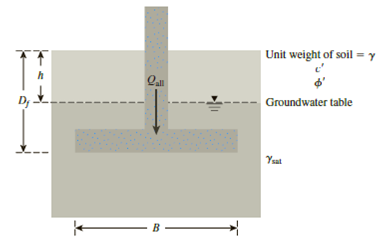Chapter 16, Problem 16.9PPrinciples of Geotechnical Enginee...

9th Edition
Braja M. Das + 1 other
ISBN: 9781305970939

Solutions

Chapter
SectionPrinciples of Geotechnical Enginee...

9th Edition
Braja M. Das + 1 other
ISBN: 9781305970939
Textbook Problem

A square footing is shown in Figure 16.18. Determine the gross allowable load, Qall, that the footing can carry. Use Terzaghi’s equation for general shear failure (Fs = 4). Given: γ = 17 kN/m3, γsat = 19.2 kN/m3, c′ = 32 kN/m3, ϕ ′ = 26 ° , Df = 1 m, h = 0.5 m, and B = 1.5 m.Figure 16.18

To determine

Find the gross allowable load (Qall) that the footing can carry.

Explanation

Given information:

The factor of safety Fs is 4.0.

The unit weight of the soil γ is 17kN/m3.

The saturated unit weight of the soil γsat is 19.2kN/m3.

The value of cohesion c is 32kN/m2.

The soil friction angle ϕ is 26°.

The depth of foundation Df is 1.0 m.

The depth of soil above water table h is 0.5 m.

The width of footing B is 1.5 m.

Calculation:

Determine the magnitude of q using the relation.

q=γ(Dfh)+γh=γ(Dfh)+(γsatγw)h (1)

Here, γ is the effective unit weight of soil and γw is the unit weight of water.

Take unit weight of water as 9.81kN/m3.

Substitute 17kN/m3 for γ, 1.0 m for Df, 0.5 m for h, 19.2kN/m3 for γsat, and 9.81kN/m3 for γw in Equation (1).

q=17(1.00.5)+(19.29.81)0.5=13.19kN/m2

Determine the ultimate bearing capacity of the soil (qu) using the relation.

qu=1.3cNc+qNq+0.4γBNγ

Here, Nc is the contribution of cohesion, Nq is the contribution of surcharge, and Nγ is the contribution of unit weight of soil

Still sussing out bartleby?

Check out a sample textbook solution.

See a sample solution

The Solution to Your Study Problems

Bartleby provides explanations to thousands of textbook problems written by our experts, many with advanced degrees!

Get Started

What is an apprenticeship?

Precision Machining Technology (MindTap Course List)

If such an e-mail came in, what would be the best action to take?

Principles of Information Security (MindTap Course List)

What is a DBMS, and what are its functions?

Database Systems: Design, Implementation, & Management

Define an object, and provide three examples.

Systems Analysis and Design (Shelly Cashman Series) (MindTap Course List)

When is a fire watch needed?

Welding: Principles and Applications (MindTap Course List)

Summarize the process you used to determine your IP address.

Enhanced Discovering Computers 2017 (Shelly Cashman Series) (MindTap Course List)